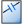ANonlinear Capacitor circuit component definition applies nonlinear behavior to the component based on the instantaneous voltage across its cell edge.

Nonlinear capacitors should be used when only time-domain results are of interest. This is because nonlinear capacitors violate the assumptions of linear system theory, making it incorrect to apply either a Fourier or discrete Fourier transform to the time-domain results. A simulation containing a nonlinear capacitor produces invalid frequency-domain results—including impedances, S-parameters, and steady-state far-zone fields—even if the time-domain results eventually decay to zero. The time-domain results are unaffected, and therefore are valid.

A nonlinear capacitor's behavior is defined by the following equation:

\begin{equation} C = C_2 + \frac{C_1 - C_2}{1 + a_1\left(\frac{ \vert V \vert - V_s}{V_0}\right)^2 + a_2\left(\frac {\vert V \vert - V_s}{V_0}\right)^4 + a_3\left(\frac {\vert V \vert - V_s}{V_0}\right)^6} \end{equation}

where

$C =$ instantaneous cell edge capacitance

$V =$ instantaneous cell edge voltage

$C_1 =$ capacitance at the cutoff voltage

$C_2 =$ infinite $\vert V \vert$ capacitance

$V_s =$ voltage magnitude

$V_0 =$ cutoff voltage. If $\vert V \vert \leq V_0$ then $C=C_1$.

$a_1$ , $a_2$ , $a_3$ are weighting coefficientsDouble-clicking on a circuit component definition in the Definitions branch of the Project Tree opens the Circuit Component Definition Editor, where a nonlinear capacitor is created by choosing Nonlinear Capacitor from the Type drop-down menu. Users should note that a nonlinear capacitor can be combined with a parallel resistor.

Rated Voltage & Current settings apply to electrostatic discharge (ESD) analysis. Both Rated Voltage and Rated Current default to infinity, but users can change either value by unchecking the associated box and entering the desired value or expression. These settings do not affect energy propagation through the component, but XF checks the voltage and current at each timestep during a simulation to see if they exceed the rated values. Users can adjust these settings to flag components that are likely to experience damage or failure during a simulation.